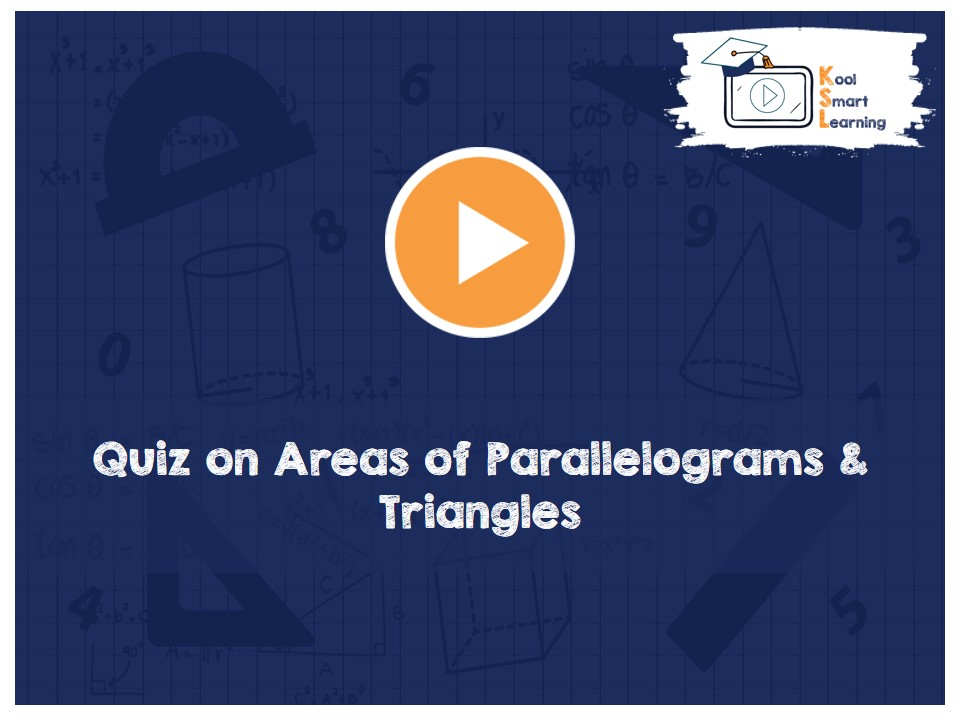At KoolSmartLearning, we intend to harness the power of online education to make learning easy.## Quiz on Areas of Parallelograms & Triangles

/  Quiz on Areas of Parallelograms & Triangles## Quiz on Areas of Parallelograms & Triangles

This video contains a quiz on the areas of parallelograms and triangles & discusses the solutions of each of the questions. The questions involve the application of the concepts and trying to find the relation between area/perimeter of different figures like areas of rectangle and perimeter with equal areas, finding areas of different figures within a parallelogram by using the corresponding theorems and more.

More Related Videos